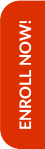Olympiads are the stepping stones to achieve better results in the competitive world that lies ahead in the life of the child. Math Olympiad examinations help students to improve their mathematical skills along with their analytical and problem solving abilities.

Hence, Olympiad Success Live has designed the course for Math Olympiad for class 3 in such a way that the foundation of the child is built up. For this, we have done great efforts in finding the tutor for class 3 Math Olympiad with relevant background and experience.

If you are interested in purchasing this course, then please Enrol Now. You will be redirected to the batch detail page, wherein you can see all the details like batch start and demo dates, fess and the registration link related to Math Olympiad for class 3 course.

##### Syllabus

Numerals, Number Name and Number Sense

• Even and odd numbers
• Comparison of numbers and arrange them in ascending and descending order
• Number names and their numerals (Up to 4-digits)
• To make greatest and smallest numbers using the given digits
• Successor and predecessor
• Roman numerals
• Place value and face value
• Indian place value chart and International place value chart

Computation operations

• Addition and subtraction of numbers (Up to 4-digits)
• Multiplication and division of numbers

Fractions

• Fraction on the number line
• Comparison of fractions
• Equivalent fractions
• Addition and subtraction of fraction with same denominator
• Addition and subtraction on the number line
• Fraction of collections of objects

Money

• Conversion of rupees into paise and vice versa
• Addition and subtraction of money
• Multiplication and division of money

Length and Weight

• Length
• Conversion of length (m-cm-km)
• Addition and subtraction of length
• Multiplication and division of length
• Weight
• Conversion of weight (mg-g-kg)
• Addition and subtraction of weight
• Multiplication and division of weight

Capacity and Quantity

• Conversion of volume and capacity (ml-l-kl)
• Addition and subtraction of volume and capacity
• Multiplication and division of volume and capacity

Time

• The clock
• Conversion of time
• Addition and subtraction of time
• Day, month and year
• Calendar

Geometry

• Definitions of line, point and line segment
• Plane figures and solid shapes
• Faces, edges and corners

Temperature

• Understanding temperature in degree Celsius
• Estimate and measure temperature in degree Celsius
• Addition and subtraction of temperature

Online classes started for English, Mathematics, Science and Reasoning for classes (3rd to 10th)